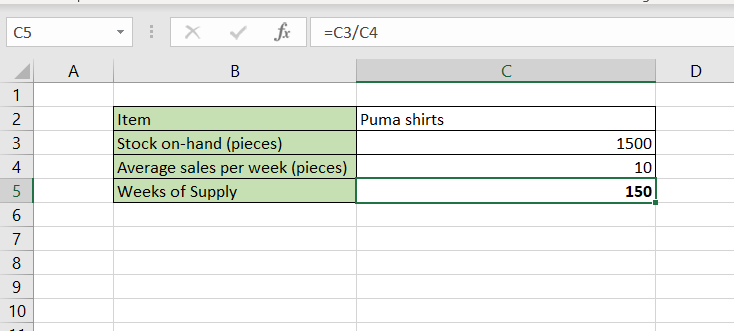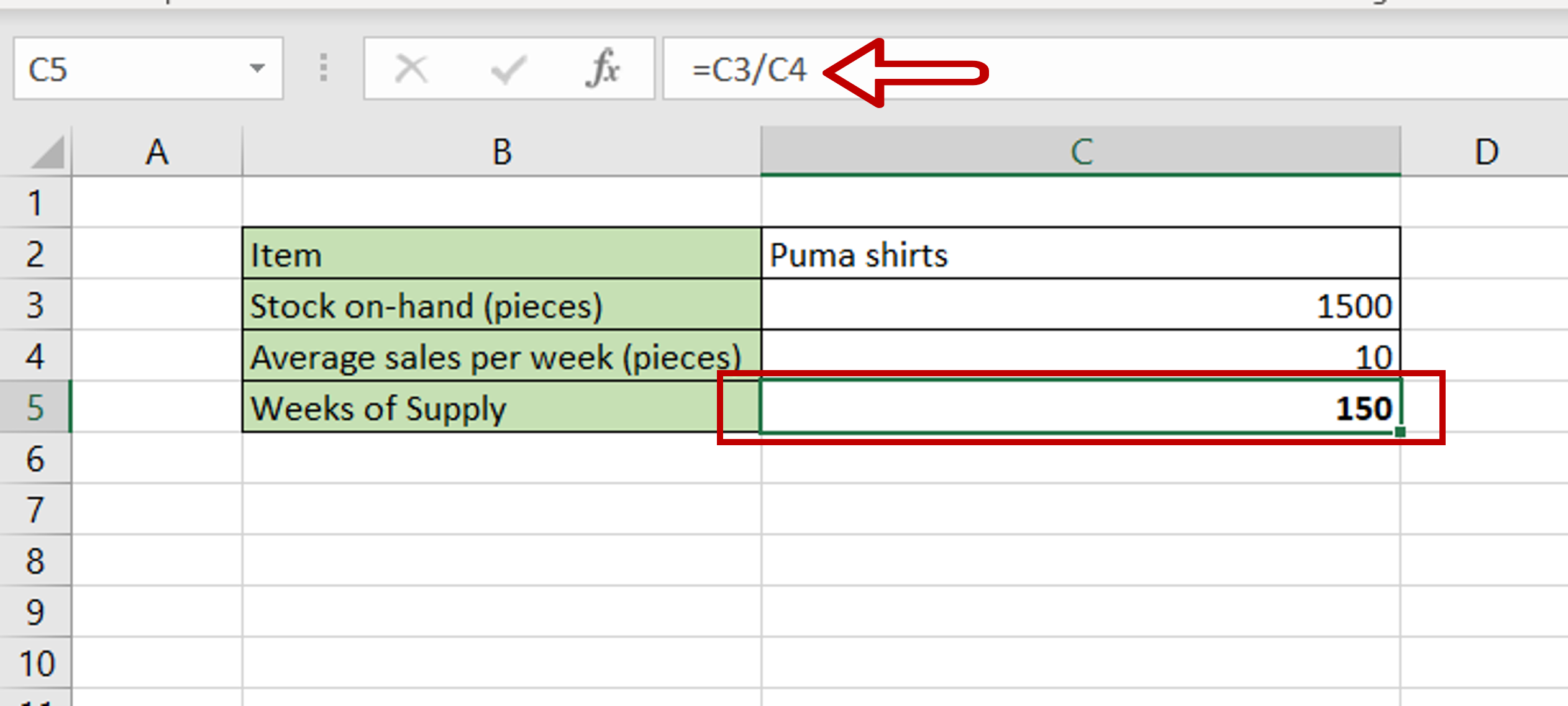# How to calculate Weeks of Supply in Excel

You can watch a video tutorial here.Excel is well-suited to manage the records of small businesses. If you keep track of your inventory in Excel, Weeks of Supply (WOS) is a metric that you will need to maintain as well. This metric tells you how many weeks your stock of a product will last, given the average rate of sale.  Excel does not have a function for this metric but it can be computed by creating a formula.

The formula for calculating the Weeks of Supply metric is:

– Weeks of Supply = Inventory on hand/Average units sold per week
>Inventory on hand is the quantity of stock you currently have
>Average sales per week is computed based on historical data

### Step 1 – Create the formula– Select the cell where the result is to appear
– Type the formula using cell references:
= Stock on-hand (pieces)/ Average sales per week (pieces)
– Press Enter